﻿ Silver Bullet for the Conceptual Comprehension of the Terms Negative Order and Fractional Order in the Field of Chemical Kinetics

### Silver Bullet for the Conceptual Comprehension of the Terms Negative Order and Fractional Order in t...OPEN ACCESSPEER-REVIEWED

## Silver Bullet for the Conceptual Comprehension of the Terms Negative Order and Fractional Order in the Field of Chemical Kinetics

R. Sanjeev1,, V. Jagannadham2, Adam A Skelton1, R. Veda Vrath3

1Department of Pharmacy, School of Health Science, University of KwaZulu-Natal, Durban, South Africa

2Department of Chemistry Osmania University, Hyderabad, India

3Department of Chemistry L N Gupta Evening College, Hyderabad, India

### Abstract

The textbook followed by freshmen engineering students (at least in reputed universities of India), is “Elements of Physical Chemistry,” by Peter Atkins and Julio de Paula. Considerable amount of chemical kinetics is dealt in this book, but negative order and fractional orders, which are fundamental for the complete comprehension of chemical kinetics, are not dealt conceptually in this book. The same is the case with other standard text books meant for freshmen undergraduate students. Many a time, even after grappling with complex problems in accounting of rate laws, students do not understand the precise conceptual meaning of these terms. The conspicuous absence of conceptual explanations of these terms in the freshmen text books has prompted us to write this article. The authors are of the opinion that, after going through this article one would definitely feel that, this article is a silver bullet for the conceptual comprehension of the terms negative order and fractional orders in the field of chemical kinetics.

• Sanjeev, R., et al. "Silver Bullet for the Conceptual Comprehension of the Terms Negative Order and Fractional Order in the Field of Chemical Kinetics." World Journal of Chemical Education 2.1 (2014): 4-7.
• Sanjeev, R. , Jagannadham, V. , Skelton, A. A. , & Vrath, R. V. (2014). Silver Bullet for the Conceptual Comprehension of the Terms Negative Order and Fractional Order in the Field of Chemical Kinetics. World Journal of Chemical Education, 2(1), 4-7.
• Sanjeev, R., V. Jagannadham, Adam A Skelton, and R. Veda Vrath. "Silver Bullet for the Conceptual Comprehension of the Terms Negative Order and Fractional Order in the Field of Chemical Kinetics." World Journal of Chemical Education 2, no. 1 (2014): 4-7.

 Import into BibTeX Import into EndNote Import into RefMan Import into RefWorks

### 1. Introduction

Let us first define order of a reaction (as described in many books): It has been found experimentally that the rate of reaction is proportional to the product of concentration of reactants, each reactant term being raised to some power. For a reactionTherefore (dx/dt) or rate α [A]α [B]β(1)

This equation 1 is known as rate law. Thus the equation which relates rate of a reaction with concentration of reactants is called rate law.

x + y = n, here ‘n’ is called the total order of the reaction. Thus, order of reaction is defined as the sum of the powers to which each of the concentration terms are raised in the rate equation. ‘x’ is known as order of the reaction with respect A, and ‘y’ is the order of the reaction with respect to B.

The constant k in the rate equation is called specific reaction rate. It is defined as the rate of reaction when the concentration of each reactant term is unity. ‘k’ is characteristic of the reaction and its magnitude depends on temperature. The units of specific reaction rate or rate constant of the reaction depends on upon the order of the reaction.

### 2. Discussion

What we described regarding the order of a reaction in the foregoing paragraphs, is a mathematical statement, and is given in many books (as previously mentioned). This does not convey the concept of the order of a reaction. In view of this, we present here the conceptual interpretation of the term “order of the reaction”. (Further, the conceptual comprehension of order of reaction is prerequisite for the understanding of fractional and negative order of reactions).

Consider the reaction of a particular order, say ‘n’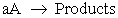(2)

For the reaction (2), rate of reaction = k[A]n

Case (i)

Let us assume that the order of the reaction (2) is one and the concentration of ‘A’ is unity.

Rate of reaction,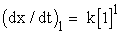(3)

Now let us suppose for the same reaction the concentration of ‘A’ is doubled. Then we get

Rate of reaction,(4)

Dividing equation (4) by (3) the ratio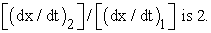Case (ii)

Now let us assume that the order of the reaction (2) is two and the concentration of ‘A’ is unity. Therefore

Rate of reaction,(5)

Now let us suppose for this second order reaction the concentration of ‘A’ is doubled. Then we get

Rate of reaction,(6)

Dividing equation (6) by (5) the ratioCase (iii)

Now let us assume that the order of the reaction (2) is three and the concentration of ‘A’ is unity. Therefore

Rate of reaction,(7)

Now let us suppose for this third order reaction the concentration of ‘A’ is doubled. Then we get

Rate of reaction,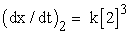(8)

Dividing equation (8) by (7) the ratioNow let us accrue the foregoing three cases i.e. (i), (ii) and (iii) and conclude analytically the conceptual interpretation of order.

When the order of the reaction is 1, and the concentration of reactant is doubled then we observe that the rate is also doubled. In the case of second order when the concentration of reactant is doubled the rate increases by four folds. And in the case of third order reactions when the concentration is doubled we observe that the rate increases by eight folds. Thus order can be conceptually perceived as a direct measure of sensitivity of reaction rate, to the changes in concentration of reactant. Third order reactions are more sensitive to the changes in concentration of reactant than those of second order. And second order reactions are more sensitive to changes in concentration of reactant than those of first order. When the reaction order is zero, it suggests that the reaction is insensitive to the changes in concentration.

Now let us consider an example where we come across negative order with respect to a particular species. The decomposition of ozone was studied by Chapman and his coworkers [2, 3], and they found the reaction to be of second order with respect to the concentration of ozone. Jahn  found the rate to be inversely proportional to the oxygen concentration, and to explain this he proposed the mechanism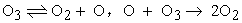The decomposition of a molecule as simple as ozone, however, must be in its second order-region except at extremely high pressures; Benson and Axworthy  therefore modified the Jahn’s mechanism as follows.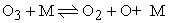; k1 and k-1 are the rate constants for the forward and backward reactions.; k2 is the rate constant for this step. Application of the steady-state principle (the rate at which the intermediate O is formed equals to the rate at which the intermediate O is removed) to the concentration of oxygen atoms gives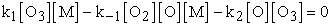and therefore by rearrangement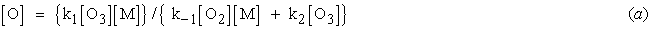The rate of decomposition of ozone is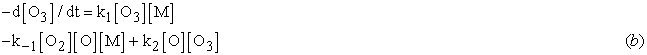and insertion of equation (a) for [O] gives rise to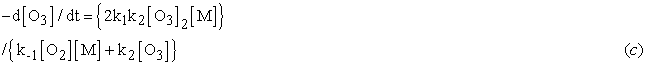The equation accounts for the fact that the rate is inversely proportional to the oxygen concentration, when sufficient oxygen is present and for the second order behavior in ozone, when oxygen is present in excess.(oxygen in excess)

Thus from equation (d) we get order with respect ozone is 2, the order with respect to oxygen is -1 and the overall order of the reaction is 1. This leads to an important query, “What does negative order with respect to oxygen mean?” To answer this query one has to extrapolate the reasoning of conceptual comprehension of first order reaction {the result of case (i)}.

Let us assume that the order of the reaction (2) is -1* and the concentration of ‘A’ is unity.

Rate of reaction,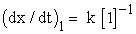(9)

Now let us suppose for the same reaction the concentration of ‘A’ is doubled. Then we get

Rate of reaction,(10)

Dividing equation (10) by (9) the ratioIn the case of a reaction of order -1, if the concentration of reactant is doubled, the rate of the reaction decreases by two times. Thus, the conceptual comprehension of negative order is explained.

If one comes across -2 order with respect to some reactant, say [A], it would suggest that, when the reactant concentration is doubled the rate decreases four fold. And if one comes across -3 order of reaction, it would mean that, when the reactant concentration is doubled the rate of the reaction decreases 8 fold.

Now let us try to understand fractional order reactions conceptually. For this purpose let us suppose the order of reaction (2) to be. and the concentration of A to be unity. Then we get

Rate,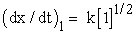(11)

And doubling the concentration of ‘A’ we get

Rate,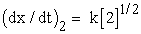(12)

Dividing equation (12) by equation (11) we get the ratio [(dx/dt)2]/[(dx/dt)1] as 1.414. Thus, when we double the concentration of A (in case of reaction with order.) the rate of the reaction increases by 1.414. Thus, the understanding of fractional order of reaction is achieved.

Let us take a familiar example of thermal reaction of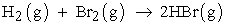The following radical chain mechanism has been proposed:

Step I Initiation: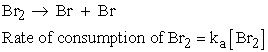Step II PropagationStep III Retardation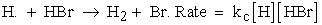Step IV TerminationThe ‘third body’, M, a molecule of an inert gas, removes the energy of recombination; the constant concentration of M has been absorbed into the rate constant kd. Other possible termination steps include the recombination of H atoms to form H2 and the combination of H and Br atoms; however it turns out that only Br atom recombination is important.

Now we establish the rate law for the reaction. The experimental rate law is expressed in terms of the rate of formation of product, HBr, so we start by writing an expression for its net rate of formation. Because HBr is formed in step 2 (by both reactions) and consumed in step 3, the net rate of formation of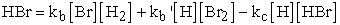(A)

We need the concentrations of intermediates Br and H, for this we set up the expressions using the steadystate approximation to both:

Net rate of formation ofNet rate of formation of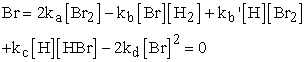The steady-state concentrations of the intermediates are found by solving these two equations and areWhen we substitute these concentrations into equation (A) we obtain

Rate of formation of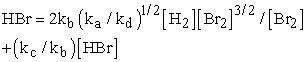Substituting k = 2kb(ka/kd)1/2 and k’ = (kc/kb’) we get

Rate of formation of(13)

For the above thermal reaction of H2 and Br2, at the very beginning of the reaction the concentration of HBr formed is significantly lower, and hence the term k’[HBr] can be neglected in the denominator of equation (13) and we can rewrite the rate law as:

Rate of formation of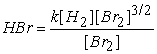(14)

And hence, order with respect to [Br2] is half.

Therefore, in such a situation as equation (14), if we double the concentration of Br2, keeping the concentration of H2 constant, then the rate of formation of HBr increases by 1.414 times (extrapolating the concept of the ratio of equation 12 and 11).

Again if we carry out the thermal reaction of H2 and Br2 under pseudo conditions where in [Br2]>>>>[H2], then [Br2]>>>>[HBr], we can neglect k’[HBr] in equation (13), we get the same rate law as equation (14), i.e. order with respect to [Br2] is 1/2.

Therefore, in such a situation as in equation (14), if we double the concentration of Br2, keeping the concentration of H2 constant, the rate of formation of HBr increases by 1.414 times (extrapolating the concept of the ratio of equation 12 and 11).

Let us take the familiar example of formation of phosgene by the reaction ofThe reaction appears to follow the following mechanism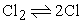rate constants: k1 and k-1rate constants: k2 and k-2rate constant: k3

The rate expression is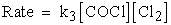In order to substitute for the intermediate [COCl], we apply the steady state approximation. Applying the steady state approximation to [COCl], we get

Net rate of formation of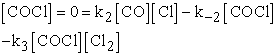(B)

from which we get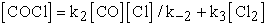Applying steady state treatment to [Cl], we get

Net rate of formation of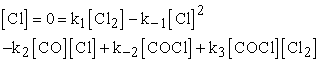(C)

Adding equations (1) and (2), and solving for [Cl] we get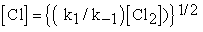Therefore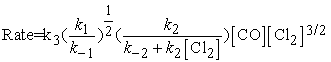If we carry out this reaction under the conditions of [CO]>>>[Cl2] and k-2>>>k3[Cl2] then above rate law is reduced to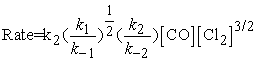Thus, the order with respect to Cl2 would be 3/2. Extrapolating the conceptual interpretation of fractional order described by taking equation (12) and equation (11), we can conclude that if the concentration of Cl2 is doubled, keeping the concentration of [CO] constant, the rate increases by 2.83 times.

Consider the acid catalyzed reaction (problem 11.26)forward & backward rate constants are k1 and k1’respectively.

HAH+ + B → BH+ + AH (slow) rate constant is k2

Rate of formation of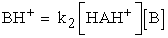HAH+ is an intermediate involved in a rapid preequilibriumRate of formation of BH+ = (k2 k1 / k1’) [HA][H+] [B]

The rate law can be made independent of [H+], if the source of H+ is the acid HA, for then H+ is given by another equilibrium.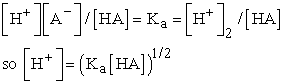Thus the deduced rate law which can be made independent of the specific term [H+] is given by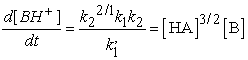(15)

It can be seen from the above rate law (15), that the order with respect to HA is 3/2. Again extrapolating the conceptual interpretation of fractional order described by equations (12) and (11), we can conclude that if the concentration of HA is doubled, keeping the concentration of [B] constant, the rate of formation of BH+ increases by 2.83 times. Thus the comprehension of fractional orders exemplified.

*Important Note: Normally, the negative order with respect to a reactant occurs, only in complex reactions in which more than one reactant is involved. It is improbable (to the best of the knowledge of authors, such situation(s) have not been encountered) in the reaction in equations like described in equation (2). But improbability should not be misconstrued as impossibility. Theoretically, reaction of the type described in equation (2) with negative order is possible. Further, to explain negative order conceptually in a lucid manner, which is comprehensible to freshman students, if one refers to the reaction of the type (2) it would be easy for the teacher and the taught.

### Acknowledgement

There isn’t a better high than watching 3 year and 5 year old kids grow. Providence has given me an endearing opportunity to watch Abubker Yimam Eshetu and Mukhtar Yimam Eshetu grow. I (R.Sanjeev) would like to dedicate this article to these two adorable neighbors and bless them that they become great Scientists of Ethiopia.

### References

  Raymond Chang. Chemistry, Ninth Edition, pp 553.In article  D. L. Chapman and H. E. Jones, “CCLIV.-The homogeneous decomposition of ozone in the presence of oxygen and other gases”, J. Chem. Soc., Vol., 97, 1910, pp. 2463-2477.In article CrossRef  D. L. Chapman and H. E. Clarke, “CLX.-The measurement of a homogeneous chemical change in a gas. (The thermal decomposition of ozone.)”, J. Chem. Soc., Vol. 93, 1908, pp. 1638-1645.In article CrossRef  Stephan Jahn, “Beitrage zur Kenntnis des Ozons” Z. Anorg. Chem., Vol.48, 1906, pp. 260-293, See also E. P. Perman and R. H. Greaves. “The Decomposition of Ozone”, Proc. R. Soc., London A, Vol. 80, 1908, pp. 353-358.In article  S.W. Benson and A.E. Axworthy, “Mechanism of the Gas Phase, Thermal Decomposition of Ozone”, J. Chem. Phys. Vol. 26, 1957, pp. 1718-1726.In article CrossRef  Peter Atkins and Julio de Paula, Elements of Physical of Chemistry; Fourth Indian Edition, pp. 278-279.In article  Peter Atkins and Julio de Paula, Elements of Physical of Chemistry; Fourth Indian Edition; pp. 283.In article